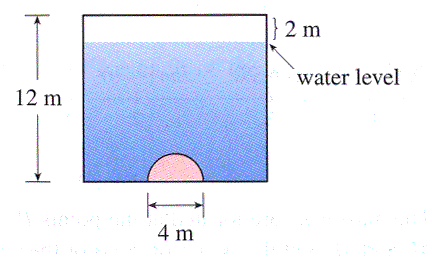A vertical dam has a semicircular gate as shown in the figure. Using the facts that the density of water is $1000\; kg/m^3$ and acceleration due to gravity is $9.8\; m/s^2,$ calculate the hydrostatic force (in N) against the gate. Make sure your answer is correct to the nearest thousand.Hydrostatic force = N